# Theory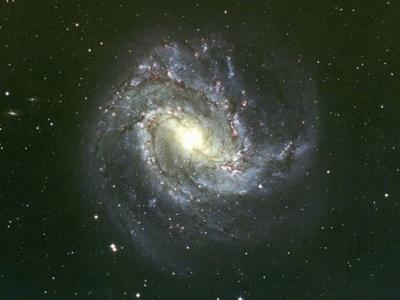## Maximum Surface Brightness

This point takes a while to explain so stay with me through this section because it's really really really important.

As shown on the Minimum Magnification page, the maximum surface brightness for extended sources, like planets and nebulae, is achieved with the minimum magnification for the telescope. Let's figure out just how bright that is.

The total gain in light that the telescope collects, over that of the eye alone, we call it GL, is shown on the Magnitude Gain page to be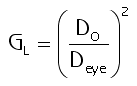Where DO is the diameter of the objective and Deye is the diameter of the eye pupil. Also shown on the Minimum Magnification page is the equation for magnification in the form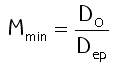Where Dep is the diameter of the exit pupil. And it is also noted on that page that the surface brightness of an object is reduced by the magnification squared, because the light is distributed over a larger area... there are a lot of things discussed on the minimum magnification page, aren't there?...

So the surface brightness of an object that you see with your eye, which I'm going to call "SBeye" since I'm not exercising much imagination, is multiplied by GL and then divided by M², resulting in the surface brightness you see in the scope, which I'm going to call "SBscope" for the same reason as above.

When the exit pupil diameter, Dep, equals the diameter of the pupil of the eye, Deye, like at minimum magnification, we have the following very interesting result for the net result in SBscope at minimum magnification: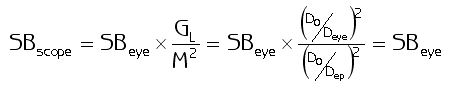What the heck is that saying? What -- that the very brightest image I can get with the scope is exactly the same brightness that I see with the naked eye??????

Yup.

Well, remember we're talking about surface brightness, meaning the brightness per unit area, or the brightness density. At the minimum magnification, which is the brightest image you can get, the surface brightness is exactly the same as what you see with your eye. Of course, the image is 30, 40, 50, even 60+ times bigger at that brightness, so it might be as much as 4000 times the total brightness, depending on the diameter of the objective. But the surface brightness never exceeds what you can see with your eye alone.

This means something else that's very important -- the images in all telescopes operating at minimum magnification have, at best*, the same surface brightness. Then we can use the brightness at minimum magnification as a reference, and since it is the maximum brightness, we can -- and we will -- consider it to be 100% brightness.

* Note - some telescopes have better optics, meaning less light loss, than others. So poorer quality scopes will not reach this "standard" brightness even at minimum magnification.

## Computing Surface Brightness from Magnification

Here comes the even bigger more important point.

As is seen in the example on the minimum magnification page, sometimes it is not only undesirable to operate the scope at minimum magnification, it's actually impossible. For telescopes with an f-ratio greater than 8, you will have a challenging time indeed trying to find the eyepiece to get you to minimum magnification, and it's not so easy to find one for f-ratios above 6.

This means that scopes are almost never operating at 100% brightness.

So... at what brightness is my scope operating? As mentioned above in passing, the surface brightness of the scope depends on the magnification. Let's consider an example.

If I'm looking at a extended source, like Jupiter, at 30 power, I can change the eyepiece out for one with half the focal length and double the power to 60x. Jupiter will then be twice the diameter in my image, and since area is a function of the diameter squared (area = π/4×D²), then when I double the diameter of Jupiter I multiply the area by 2² = 4. The telescope is still collecting the same light, which is now spread out over 4 times the area, so the surface brightness will drop by a factor of 4.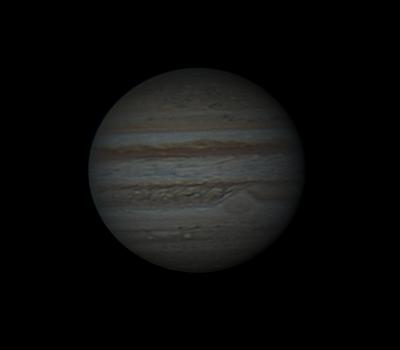Low Magnification High Magnification

Since Mmin is the magnification that gives you 100% brightness, and any increased magnification M will reduce your brightness as M², then you can find the surface brightness SB = (Mmin/M)². Simple. Keep reading.

## Computing Surface Brightness from Eyepiece Focal Length

As shown on the Minimum Magnification page, the maximum eyepiece focal length to get your minimum magnification is simply fe-max = 7×fR, where fR is the f-ratio of the scope. Since magnification M = fO/fe then we can substitute into the surface brightness equation to compute the surface brightness from the eyepiece that we are using.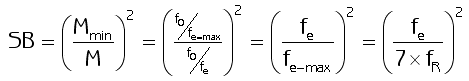Also simple. But there is a still better, simpler way to find the surface brightness, using the exit pupil...

## The Exit Pupil and Scope Performance

### Computing Surface Brightness from the Exit Pupil

You wouldn't think that the exit pupil, the diameter of the light beam leaving the eyepiece, would provide the magic key to the performance of the scope, but check this out:

Substituting the calculation of Mmin from DO we get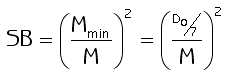Also from the Minimum Magnification page we have that the exit pupil Dep = DO/M, so we can replace DO/M in the surface brightness equation with Dep and we get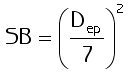Given this equation, it’s actually pretty easy to find the surface brightness from the exit pupil since 7˛ = 49, or about 50 = 100/2. Then SB = Dep˛/7˛ = 2×Dep˛/100, so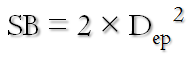is the surface brightness in percent. Seriously. Take the exit pupil, square it, double it, and you've got it.

Well, heck -- you can just knock that one out in your head... for example with an exit pupil of 5mm, then 5˛ = 25, double it and you get 50, so surface brightness with a 5mm exit pupil is 50% of maximum. If Dep is 3mm then 3˛ = 9, double it gives you 18 so the surface brightness is 18% of maximum and so forth. Just another good reason to learn those two simple exit pupil equations: Dep = DO/M and Dep = fe/fR.So we have a one-to-one correspondence between surface brightness and the exit pupil. If I define a specific exit pupil, I have also defined exactly where I am on the brightness scale, totally independent of the scope.

That means the exit pupil gives me the same information that the surface brightness does, without calculating squares of anything. The exit pupil gives me a simple way of defining and transferring settings from one scope to another, as follows:

• Once the proper settings are found to capture an image on a scope, determine the exit pupil that was used as Dep = fe/fR or Dep = DO/M.
• Calculate the magnification and/or eyepiece focal length for the second scope using M = DO/Dep and fe = Dep×fR.

Check out "The Power of the Exit Pupil" example below to see how easily you can solve problems this way.

*Another Note - here my definition of surface brightness "SB" is on a relative scale with the eye set to be the reference 100%. There is another definition of surface brightness in terms of magnitudes per square arc second (mpsas), defined with the formula S = m + 2.5×log10 A. Here m is the magnitude (total brightness) of the object and A is its area in arc seconds squared. There's a good Wikipedia article on the subject here.

### Exit Pupil and the Resolving Power of the Eye

You may have noticed that your eyesight is sharper in daylight than it is in the dark. This is not just some sort of illusion, it is a real effect based on the physics of your eye.

This is because the lens of the eye is part of your body, and it's adjustable -- it's not a computer-generated, rigid lens with mathematically perfect surfaces all the way from the center to the edge. Its shape is good (spherical) at the center, and starts to deviate from "perfection" toward the edges.

The non-spherical shape, or "spherical aberration" combines with an effect called "chromatic aberration", which means different colors passing through the lens focus at slightly different points. Like spherical aberration, chromatic aberration also is more pronounced toward the edges of the lens.

The larger the exit pupil, the more of the lens is used to focus the light, and the more the deviation from "perfect" causes errors in focusing the light. So at a 7mm exit pupil, you get the highest surface brightness, but not the sharpest focus in the eye.

Meanwhile the smaller the exit pupil, the more the diffraction limit of the pupil impedes the resolving power of the eye. So there is an optimum point where the combined effects of spherical aberration, chromatic aberration, and diffraction effects reach a minimum.

It turns out that an exit pupil of about 2-3mm (2.4mm to be precise) is the optimum point for maximizing the resolving power of the eye. This is about where theory would put it, and it has been confirmed by observational studies.

### Exit Pupil as a Universal Performance Scale

Let's see how the one-to-one correspondence between the exit pupil and brightness scale, and the dependence of the optimum resolution on exit pupil, can be used to define a performance scale for the scope based on the diameter of the exit pupil, Dep. Because the exit pupil is a scope-independent reflection of surface brightness and optimum resolution, this is a universal performance scale for all scopes.

For this interesting little exercise, we will use the fact that both magnification, M , and the focal length of the eyepiece, fe, can also be determined by the exit pupil as follows: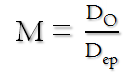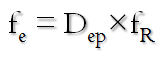Then we have the following four key reference points on our performance scale:

Maximum magnification: Dep = DO/Mmax = DO/DO = 1 mm. This might be a bit of a surprise: at max magnification, where the scope's resolution matches your eye's resolution, the exit pupil is exactly 1 mm. Also it gets smaller as you go to higher power, so if you can see the Airy disk the exit pupil is less than or equal to 1mm. Here we have a magnification of DO, an eyepiece focal length of fR, and a surface brightness of SB(%) = 2×Dep˛ = 2×1˛ = 2%.

Optimum magnification: we will assume the optimum is at 2mm, as this actually is closest to the true optimum (2.4mm), is an easy point to remember and simple for mental calculations. Bear in mind, though, that anywhere on the range between 2 and 3mm pretty much hits it. Then we have M = DO/Dep = DO/2, fe = Dep×fR = 2×fR, and a surface brightness of SB(%) = 2×Dep˛ = 2×2˛; = 8%. Note that this optimum is at half maximum magnification.

Maximum Brightness: We found brightest image, which also has the widest field of view, by setting the magnification to give us an exit pupil of 7 mm -- the minimum magnification we will want to use. At this point we have a magnification of M = DO/Dep = DO/7, eyepiece focal length fe = 7×fR, and a surface brightness of 100%.

Wide Field: by backing down from a 7mm exit pupil to 5mm, we arrive at a point that is half maximum brightness, resulting in an eyepiece focal length fe = 5×fR that sometimes is more available than the maximum eyepiece focal length. This point, with 50% max brightness and 70% of widest possible field of view (so we can still call it "wide field"), represents a good compromise in brightness vs. magnification for deep-sky observation.

To these four critical points we will add one additional point, an "Extra-high" resolution point, introduced because max magnification brings the scope's resolving power to where your eye can just see it. We can push the scope a little beyond its performance range at the high power end, to make the detail a bit easier to see.

As you go past the limit of the scope's performance you can see the effect, in the form of progressively greater rounding or blurring of edges. We therefore will stop at 50% higher magnification, or 1.5×Mmax = 1.5×DO, before the blurring (not to mention the image darkening) becomes objectionable.

Setting an extra-high magnification M = 1.5×Mmax leads to a Dep = DO/M = DO/(1.5×DO) = 2/3mm.

Then the dynamic range for the exit pupil is fundamentally from 1 to 7 mm, with three additional reference points noted for a total of 5 points on the scale. These are points to consider when selecting a set of eyepieces for use with a given scope:

 Maximum Brightness Dep = 7mm Half-Maximum Brightness Dep = 5mm Half-Maximum Magnification Dep = 2mm Maximum Magnification Dep = 1mm Extra-High Magnification Dep = 2/3mm

This is what the resulting universal performance range for telescopes looks like: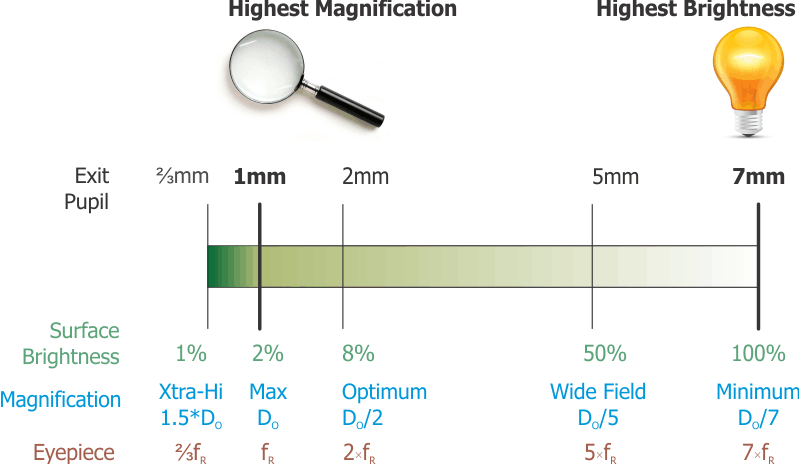• When I'm looking for faint objects, particularly when I'm using a filter, I want lots of brightness and will work at the bright end of the range (large exit pupil).
• If I have a dark sky I can afford to go with the brightest eyepiece, but if I have a light-polluted sky, or a difficult target, or both, I might back off a bit to a somewhat smaller exit pupil... 4-5mm rather than 7mm. By increasing the magnification, I spread out the background sky glow, and that can improve the contrast with the faint target. Neat trick to remember.
• If I want to split a double, I will work at the dark, high-power end of the range (small exit pupil). Remember that stars don't start to get darker until I exceed the maximum magnification of the scope, or the maximum allowed by the atmospheric seeing (usually about 150-200X). So increased magnification really improves star contrast against background sky glow.
• This performance range assumes that the f-ratio will permit it. In practice, eyepieces over 40mm are hard to find and above 56mm virtually don't exist. Therefore, for all practical purposes, scopes with an f-ratio over 6 are limited on the bright end of their performance range to an exit pupil of about 40÷fR.
• If I’m a photographer, I can compensate for low brightness with long exposures, so a low f-ratio is less important than optical perfection. Then I may go with a longer focal length in the objective, which is easier to get relatively free of artifacts (chromatic & spherical aberrations).

## Comparing One Scope to Another

### Comparison Based on Diameter

Since SB = 2×Dep², then if we want the same surface brightness we need to keep Dep the same.

We have a way to find Dep using the scope diameter DO and magnification M: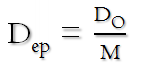Then to keep Dep the same we need to keep the ratio of scope diameter to magnification the same.

That means:

1. If we go from a smaller scope to a larger scope we can get the same surface brightness at higher magnification. For example, if we go from a 4" scope to an 8" scope, we've doubled the scope diameter (DO) so we can get the same surface brightness at double the magnification.
2. If we go from a larger scope to a smaller scope, we need to reduce the magnification to get the same surface brightness. We change the magnification by the same factor that we changed the scope diameter. So for example if we're going from an 8" scope to a 4" scope, we changed by a factor of 2 so the magnification will have to be reduced by a factor of 2 to keep the same surface brightness.
3. A larger scope at the same magnification will give a higher surface brightness. Since SB goes as Dep², surface brightness will increase with the scope diameter squared. That means when we go from that 4" scope to the 8" scope, twice the diameter, then at the same magnification we will see 2² = 4 times the surface brightness.

### Comparison Base on f-Ratio

We still have SB = 2×Dep², and if we want the same surface brightness we're still trying to keep Dep the same.

In this case we will work off the other exit pupil formula, which uses the eyepiece focal length and the f-ratio.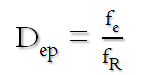Now we are looking at the effect that a different f-ratio will have, and we can see that to keep Dep the same we need to keep the ratio of the eyepiece focal length to the f-ratio the same.

That means:

1. If we go from a scope with a larger f-ratio to one with a smaller f-ratio, we can get the same surface brightness with a shorter focal length eyepiece. For example, if we are using an f/10 scope with a 20mm eyepiece and switch to an f/5 scope, we've cut the f-ratio in half so we would use a 10mm eyepiece to get the same surface brightness in the image.
2. If we go from a scope with a smaller f-ratio to one with a larger f-ratio, we need to increase the focal length of the eyepiece (moving back from the image plane and reducing magnification) to get the same surface brightness. What is interesting about these equations is that we only need to know the f-ratio of the scope to determine the eyepiece that will hit our target surface brightness.
3. A scope with a smaller f-ratio using the same eyepiece will give a higher surface brightness. Since SB goes as Dep², surface brightness will increase as the reduction in the f-ratio squared. That means when we go from that f/10 scope to the f/5 scope, half the f-ratio, then with the same eyepiece we will see 2² = 4 times the surface brightness.

# Practice

## Some Basic Examples

### My ETX 90 RA

This is a 90mm f/13.9 scope.

• then the minimum magnification is DO÷7 = 90÷7 = 12.8 ≈ 13
• the eyepiece required to get that magnification is 7×fR = 7×13.9 = 97mm – which I won’t find, and for that matter I don’t want to because magnification of 13x is about what my binoculars are doing. So I (or anyone else) would never be working at that level with this scope.
• let's say we will go with a routine value for a long-focus eyepiece, like 40mm, and I'll work the problem as I normally would:
• Find the exit pupil for this eyepiece:
Dep = fe÷fR = 40÷13.8 = 2.9mm (call it 3).
• Find the magnification with this eyepiece:
M = DO÷Dep = 90÷3 = 30 (decent)
• Find the surface brightness with this eyepiece:
SB = 2×Dep˛ = 2×3˛ = 18%
Note that since this is about the longest focal length eyepiece I can get, this is about the brightest I can get the image in this scope.
• for fun, let's see where we are with the 26mm eyepiece that came with the scope:
• Find the exit pupil for this eyepiece:
Dep = fe÷fR = 26÷13.8 = 1.9mm
• Find the magnification with this eyepiece:
M = DO÷Dep = 90÷1.9 = 47.8
• Find the surface brightness with this eyepiece:
SB = 2×Dep˛ = 2×1.9˛ = 7.2%
Pretty dim.

I just dug up the biggest aperture scope I can find looking through the catalogs on my desk. This is a 406.4mm diameter f/4.5 scope.

• then the minimum magnification is 406.4÷7 = 58 -- which is not a bad magnification value at all.
• the eyepiece required to get that magnification is 7×4.5 = 31.5mm – where a 32mm eyepiece is a common size, albeit toward the big end of the spectrum. So it is perfectly possible to operate this scope at 100% brightness.
• the scope comes with a 26mm eyepiece so let's analyze that as we did above:
• Find the exit pupil for this eyepiece:
Dep = fe÷fR = 26÷4.5 = 5.8mm (much bigger than the ETX)
• Find the magnification with this eyepiece:
M = DO÷Dep = 406.4÷5.8 = 70
• Find the surface brightness with this eyepiece:
SB = 2×Dep˛ = 2×5.8˛ = 67.3%
• which means that out of the box this scope is operating at a surface brightness that is about 10 times the brightness of my ETX at higher magnification.

So that very short f-ratio makes this a very bright, sometimes called a "fast", scope -- coupled with the large diameter this scope is a killer for deep-sky exploration.

## Comparison of the Two Scopes

### Both with the Same Eyepiece

Both of these scopes come with the same focal length eyepiece -- 26mm -- so how do they compare based on that?

With the same eyepiece, the surface brightness of the two scopes goes with the ratio of f-ratio's squared, so we get [fR1÷fR2]˛ = [13.9÷4.5]˛ = 9.54. This is telling us that the Lightbridge is 9.54 times brighter than the ETX with the same eyepiece, consistent with what we found above, but computed more directly.

### Both with the Same Magnification

So presuming we can find the appropriate eyepieces to get the two scopes operating at the same magnification, or close enough, how would the two image brightnesses compare?

At the same magnification, the surface brightness of the two scopes goes with the ratio of the scope diameters squared, so we get [DO2÷DO1]˛ = [406.4÷90]˛ = 20.4. The LightBridge would show the image at the same magnification at 20 times the brightness of the ETX 90. Yikes.

Before we continue beating up on my poor little ETX, I would hasten to point out that it cost about a quarter of what the LightBridge would set me back, and the ETX is highly portable. But for image quality, it simply has no chance against the much bigger scope.

## The Power of the Exit Pupil

I'm going to show you how great the exit pupil is by way of, what turns out to be, a real example.

### Statement of the Horse Head Nebula Problem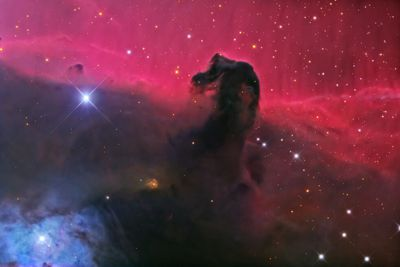We will suppose I'm out observing with a friend who has a 15" f/4.5 Obsession telescope, and that I'm using an Orion 8" f/6 Dobsonian. My friend is able to show me the Horse Head Nebula with his Obsession telescope using a Televue 22mm eyepiece. What should I use in the Orion scope to also be able to see this nebula?

### Solving It with the Exit Pupil

So the exit pupil my friend was using on his Obsession scope is Dep = fe/fR = 22/4.5 = 4.9 ≈ 5mm.

Then the eyepiece focal length for the Orion is fe = Dep×fR = 5×6 = 30mm.

Easy-peasy.

Of course, at the same brightness, the 8" scope will be operating at lower magnification than the 15" -- to be precise it will be set to 8/15, or about half, the magnification.

By the way, this example is based on real experience, although for simplicity I left out the fact that a nebular filter is also used to see the Horse Head. It was Barbara Wilson of the Houston Astronomical Society who discovered that an exit pupil of 5mm, along with using a nebular filter, is the secret to seeing the Horsehead Nebula.

## Questions

Your questions and comments regarding this page are welcome. You can e-mail Randy Culp for inquiries, suggestions, new ideas or just to chat.
Updated 28 August 2019